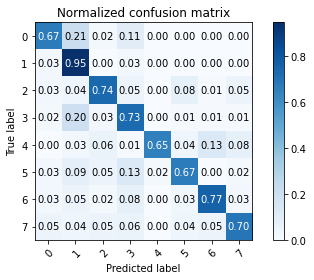# How to assign labels in confusion matrix?

Hi there I am using numerical labels so the confusion matrix looks like thiswhat to do to make this labelling as text.
I am using the code below for confusion matrix.

``````def plot_confusion_matrix(ts_labels_emotion, y_pred, classes,
normalize=False,
title=None,
label_encoder={0: 'Neutral', 1: 'Calm', 2: 'Happy',3:'Sad',3:'Angry',4:'Fear',5:'Disgust',6:'Surprised'},
cmap=plt.cm.Blues):
"""
This function prints and plots the confusion matrix.
Normalization can be applied by setting `normalize=True`.
"""
if not title:
if normalize:
title = 'Normalized confusion matrix'
else:
title = 'Confusion matrix, without normalization'

# Compute confusion matrix
cm = confusion_matrix(ts_labels_emotion, y_pred)
# Only use the labels that appear in the data
classes = unique_labels(y_pred)
if normalize:
cm = cm.astype('float') / cm.sum(axis=1)[:, np.newaxis]
print("Normalized confusion matrix")
else:
print('Confusion matrix, without normalization')

print(cm)

fig, ax = plt.subplots()
im = ax.imshow(cm, interpolation='nearest', cmap=cmap)
ax.figure.colorbar(im, ax=ax)
# We want to show all ticks...
ax.set(xticks=np.arange(cm.shape),
yticks=np.arange(cm.shape),
# ... and label them with the respective list entries
xticklabels=classes, yticklabels=classes,
title=title,
ylabel='True label',
xlabel='Predicted label')

# Rotate the tick labels and set their alignment.
plt.setp(ax.get_xticklabels(), rotation=45, ha="right",
rotation_mode="anchor")

# Loop over data dimensions and create text annotations.
fmt = '.2f' if normalize else 'd'
thresh = cm.max() / 2.
for i in range(cm.shape):
for j in range(cm.shape):
ax.text(j, i, format(cm[i, j], fmt),
ha="center", va="center",
color="white" if cm[i, j] > thresh else "black")
fig.tight_layout()
return ax

np.set_printoptions(precision=2)

``````

But I think this label encoder is not working. Any suggestions. @ptrblck sir can you plz suggest anything here, how to change this number into text labels in plotting confusion matrix.
Regards

This question seems more `matplotlib`-related so, you might generally get a faster and better answer in their discussion boards or e.g. StackOverflow.
That being said, you can use `ax.set_xticklabels(labels)` to set the labels for an axis.
Based on your code snippet, I guess you might need to modify `xticklabels=classes, yticklabels=classes`.

completely unrelated, but what `fmt` stands for?

Most likely it stands for “format” and is used to format the text.

1 Like

@ptrblck Ya i think so. but still I didt get the answer. Tried in that forum tooNow I am thinking to use photoshop kinda thing in writing paper.

Your code seems to work and is using rotated text labels:

``````x = np.random.uniform(0, 1, (10, 10))

fig, ax = plt.subplots()
im = ax.imshow(x, interpolation='nearest', cmap=plt.cm.Blues)
ax.figure.colorbar(im, ax=ax)

classes = ['label{}'.format(i) for i in range(10)]
title = 'confusion matrix'
# We want to show all ticks...
ax.set(xticks=np.arange(x.shape),
yticks=np.arange(x.shape),
# ... and label them with the respective list entries
xticklabels=classes, yticklabels=classes,
title=title,
ylabel='True label',
xlabel='Predicted label')

# Rotate the tick labels and set their alignment.
plt.setp(ax.get_xticklabels(), rotation=45, ha="right",
rotation_mode="anchor")
``````1 Like

Thank you sir, You saved me
Big Thanks
Regards

Good to hear it’s working, but I was really just reusing your code.A huge thanks for this discussion. As a newbie I was very interesting to read

1 Like